EDIT MAIN
##### Welcome to 5th Grade Math.

Make sure your student is bringing their A.R. Folder and take home folder to class daily. All needed information will be sent home in the take home folder daily.

2019-2020
Weekly Study Guides & Vocabulary will be sent home on Monday's!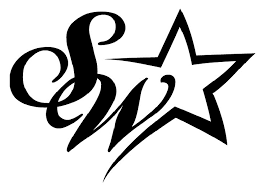Each week students will go over 4 different domains in multiple settings : independent practice, collaborative work, and teacher led note-taking. Each domain has a new skill level that students will need to master weekly. Although the TEKs are filtered back in each week; the concept is new information that the students are learning. You will receive test taking skills every Monday that will help students identify how the test will look on Friday.

Week 1 (Sept. 30- Oct.4):

Skills: Numerical Patterns

Review:Decimals and Fraction Review

Concept: Number Theory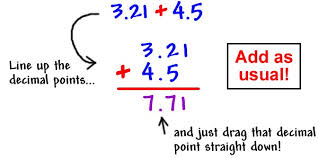Week 2(Oct. 7 - Oct.11):

Skills: Classifying Triangles

Review:Computation (Mixed Practice)

Concept: Simplifying Numerical Expressions (Order of Operations Part 1)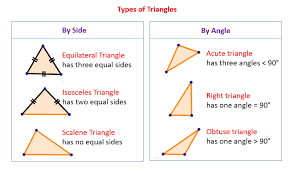Week 3(Oct. 14 - Oct. 18):

Skills: Fraction Review

Review: Rounding and Estimation, Addition and Subtraction

Concept: Simplifying Expressions (Order of Operations Part 2)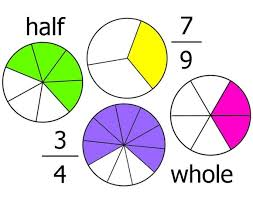Week 4(Oct. 21 - Oct. 25):

Skills: Data Analysis and Graphs

Review: Multiplication, 2-Digit x 2-Digit

Concept: Perimeter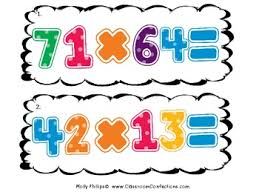Week 5 (Oct. 28 - Nov. 1):

Skills: Numerical Patterns

Review: Multiple Step Problems

Concept: Area and Perimeter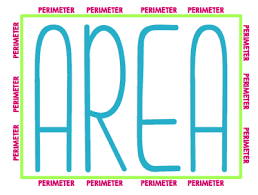Week 6(Nov. 4 - Nov. 8): Review Week!

##### 2nd 6 Weeks Vocabulary!

Week 1:

• Factors- a number that is multiplied by another number to find a product. (Example: The factors of 6 are 1,2,3, and 6.)
• Multiples- The product of a given number and any whole number. (Example: The multiples of 5 include 5,10,15,20….)
• Factor Tree- A graphic organizer listing the factor pairs of a given number.
• Factor Pairs- Two numbers whose product is a given number.
• Prime Factorization- To write a composite number as a product of its prime factors.
• Common Factors- A number that is a factor of two or more numbers. (For example: The factors of 6 are 1,2,3, and 6. The factors of 9 are 1,3,9. Common factors of 6 and 9 are 1 and 3.)
• Greatest Common Factor- The largest factor that two or more numbers have in common.
• Even Numbers- A whole number that is divisible by 2.
• Odd Numbers- A number that is not divisible by 2; such as a number has 1,3,5,7,9 in the ones place..
• T-chart: A graphic organizer listing the factor pairs of a given number.

Week 2 & Week 3:

• Order of Operations- The order in which the mathematical process is completed.
• Expression- A mathematical combination of numbers, operations, and/or variables.
• Equation- A number sentence that uses the equal sign to show that two expressions are equal.
• Value- How much a digit is worth.
• Parentheses- symbols ( ) used to group part of a mathematical expression or equation.
• Brackets- symbols [ ] used to group part of a mathematical expression or equation.
• Simplify- To write an expression, equation, or fraction in lowest terms.
• Numerical Expression- A mathematical combination of numbers, operations, and/or variables.
• Quotient- The answer to a division problem.
• Product- The answer to a multiplication problem.
• Difference- The answer to a subtraction problem.
• Factor- a number that is multiplied by another number to find a product. (Example: The factors of 6 are 1,2,3, and 6.)
• Divisor- The number by which another number is divided.
• Dividend- The number to be divided in a division problem.
• Remainder- The number left over after dividing into equal groups.
• Equivalent- The same value or amount.
• Division- The operation of making equal groups to find the number in each group or to find the number of equal groups.
• Divisible- When one number can be divided by another number with no remainder. (For example: 21 is divisible by 3 and 7.)

Week 4:

• Perimeter- The distance around a closed, two-dimensional figures.
• Dimensions- A measurable length, depth, or height.
• Squares- A 4 sided figure with 4 congruent sides, 4 right angles, and 4 vertices.
• Rectangles- A 4 sided figure with 4 sides, 4 right angles, and 4 vertices.
• Length- The measurement of distance between two points.
• Increments- An increase or addition, especially one of a series on a fixed scale.
• Equation- A number sentence that uses the equal sign to show that two expressions are equal.
• Expression- A mathematical combination of numbers, operations, and/or variables.
• Numerator- The top number of a fraction.
• Denominator-The bottom number of a fraction.
• Line Segment- Part of a line between two endpoints.
• Units of Measure- A definite magnitude of a quantity
• Proper Fractions- A fraction with a numerator less than the denominator.
• Decimal Number- A number that uses a decimal point to show tenths, hundredths, and thousandths.
• Decimal Point- The dot used to separate the ones place from the tenths place in a decimal number.

Week 5:

• Area- The number of square units needed to cover a surface without gaps or overlaps.
• Perimeter- The distance around a closed, two-dimensional figure.
• Dimensions- A measurable length, depth, or height.
• Congruent- Having the same size and shape
• Surface Area-
• Line- A set of points that from a straight path that goes in opposite directions without ending.
• Parallel lines- Lines that never meet or intersect; always the same distance apart.
• Perpendicular Lines- Lines intersecting to form right angles.
• Intersecting Lines- Lines that meet or cross at a common point.
• Polygon- A closed figure made of 3 or more straight line segments.
• Volume- The number of cubic units needed to fill the space occupied by a solid.
• Angle- The figure formed by two rays that extended from a common endpoint. {right, obtuse, acute}
• Triangle- A polygon with 3 sides, 3 angles, and 3 vertices.{ Isosceles, equilateral ,right, and scalene.}
• Hierarchy- An arrangement or grouping that shows how items in one category relate to items in another category.
• Expression- A mathematical combination of numbers, operations, and/or variables.